# Current Divider Rule: What is it? Formula, Derivation & Examples

Contents

## What is a Current Divider?

A current divider is defined as a linear circuit that produces an output current that is a fraction of its input current. This is achieved through the connection of two or more circuit elements connected in parallel, the current in each branch will always divide in such a way that the total energy expended in a circuit is minimum.

In other words, in a parallel circuit, the supply current splits into a number of parallel paths. It is also known as the “current divider rule” or “current divider law”.

A parallel circuit is often called current divider in which terminals of all the components are connected in such a way that they share the same two end nodes. These result in different parallel paths and branches for the current to flow through it.

Hence current in all the branches of the parallel circuit is different but voltage is the same across all the connected paths. i.e.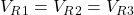…. etc. Therefore, there is no need to find the individual voltage across each resistor which allows the branch currents to be easily found by KCL (Kirchhoff’s Current Law) and ohm’s law.

Also, in the parallel circuit, the equivalent resistance is always less than any of the individual resistances.

## Current Divider Formula

A general formula for a current divider is given by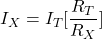Where,

•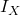= Current through any resistor in the parallel circuit =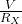•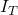= Total current of the circuit =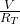•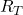= Equivalent resistance of the parallel circuit
•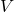= Voltage across the parallel circuit =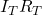=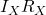(as the voltage is same across all the components of the parallel circuit)

In terms of impedance, the formula for a current divider is given by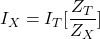In terms of admittance, the formula for a current divider is given by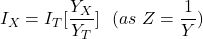### Current Divider Formula for RC Parallel Circuit

Apply current divider rule to the above circuit, the current through the resistor is given by,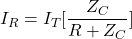Where,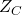= Impedance of the capacitor =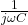Thus we get,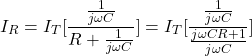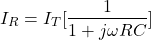## Current Divider Rule Derivations

Consider a parallel circuit of two resistors R1 and R2 connected across a supply voltage source of V volts.

Assume that the total current entering the parallel combination of resistors is IT. The total current IT divides into two parts I1 and I2 where I1 is the current flowing through the resistor R1 and I2 is the current flowing through the resistor R2.

Therefore, the total current is

(1)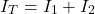or

(2)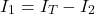or

(3)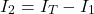Now, when two resistors are connected in parallel, the equivalent resistor Req is given by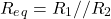(4)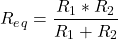Now according to the ohm’s law i.e.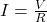, the current flowing through the resistor R1 is given by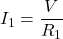(5)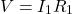Similarly, the current flowing through the resistor R2 is given by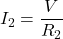(6)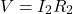compare equation (5) and (6) we get,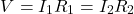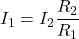Put this value of I1 into equation (1) we get,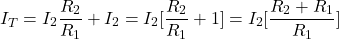(7)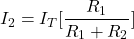Now put this equation of I2 into equation (2), we get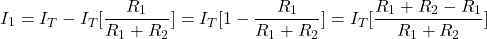(8)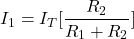Thus, from equation (7) and (8) we can say that the current in any branch is equal to the ratio of opposite branch resistance to the total resistance value, multiplied by the total current in the circuit.

In general,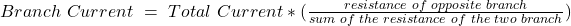## Current Divider Examples

### Current Divider for 2 Resistors in Parallel With Current Source

Example 1: Consider two resistors 20Ω and 40Ω are connected in a parallel with a current source of 20 A. Find out the current flowing through each resistor in the parallel circuit.

Given data:      R1 = 20Ω, R2 = 40Ω and IT = 20 A

• Current through resistor R1 is given by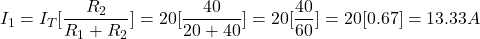(9)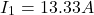• Current through resistor R2 is given by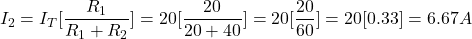(10)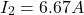Now, add equation (9) and (10) we get,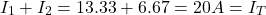So that according to Kirchhoff’s Current Rule, all the branches current is equal to the total current. Thus, we can see that the total current (IT) is divided according to the ratio determined by the branch resistances.

### Current Divider for 2 Resistors in Parallel With Voltage Source

Example 2: Consider two resistors 10Ω and 20Ω are connected in a parallel with a voltage source of 50 V. Find out the magnitude of total current and the current flowing through each resistor in the parallel circuit.

Given data:      R1 = 10Ω, R2 = 20Ω and V = 50 V

• The equivalent resistance of the parallel circuit is given by,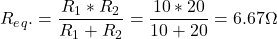• According to ohm’s law, the total current flowing through the parallel circuit is given by,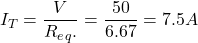• Now, according to current divider formula the Current through resistor R1 is given by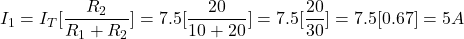(11)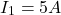• Current through resistor R2 is given by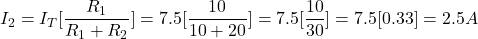(12)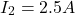Now, add equation (11) and (12) we get,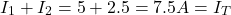So that according to Kirchhoff’s Current Rule, all the branches current is equal to the total current. Thus, we can see that the total current (IT) is divided according to the ratio determined by the branch resistances.

### Current Divider for 3 Resistors in Parallel

Example 3: Consider three resistors 20Ω, 30Ω, and 40Ω are connected in a parallel form a current divider circuit as shown below. The circuit is connected with a 100V supply. Find out the total current and the current flowing through each resistor in the parallel circuit using the current division rule.

Given data:      R1 = 20Ω, R2 = 30Ω, R3 = 40Ω and V = 100 V

• The equivalent resistance of the parallel circuit is given by,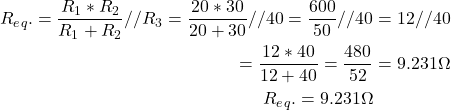• According to ohm’s law, the total current flowing through the parallel circuit is given by,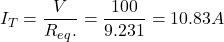• Now, according to current divider formula, the current through resistor R1 is given by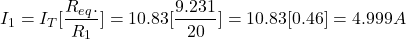(13)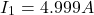• Current through resistor R2 is given by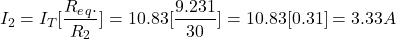(14)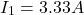• Similarly, current through resistor R3 is given by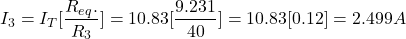(15)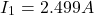Now, add equation (13), (14) and (15) we get,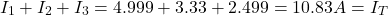So that according to Kirchhoff’s Current Rule, all the branches current is equal to the total current. Thus, we can see that the total current (IT) is divided according to the ratio determined by the branch resistances.

Note that from all the three examples we can say that smaller the resistance having the greatest share of the current and vice versa. Also, the supply current is equal to the sum of individual branch currents.

### When You Can Use the Current Divider Rule

You can use the current divider rule in the following circumstances:

• The Current divider rule is used when two or more circuit elements are connected in parallel with the voltage source or the current source.
• The Current divider rule can also be used to determine individual branch currents when the total circuit current and the equivalent resistance are known.
• When two resistors are connected in a parallel circuit, the current in any branches will be a fraction of the total current (IT)). If both the resistors are of equal value, then the current will divide equally through both the branches.
• When three or more resistors are connected in parallel then the equivalent resistance (Req.) is used to divide the total current into fractional currents for each branch in the parallel circuit (See example 3).

Want To Learn Faster? 🎓
Get electrical articles delivered to your inbox every week.
No credit card required—it’s 100% free.